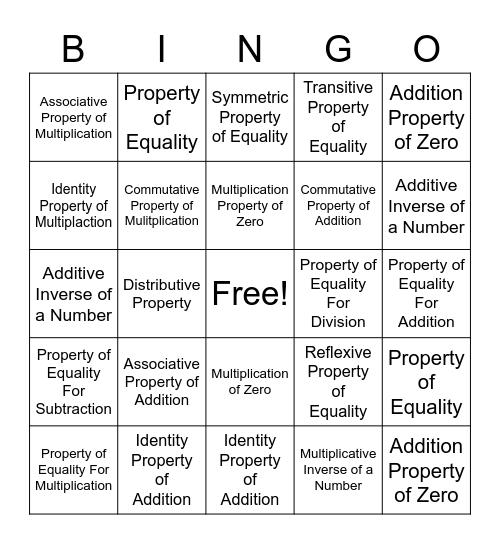# Properties of MathematicsThis bingo card has a free space and 24 words: Associative Property of Addition, Identity Property of Addition, Multiplication Property of Zero, Property of Equality For Multiplication, Addition Property of Zero, Associative Property of Multiplication, Identity Property of Multiplaction, Property of Equality, Property of Equality For Division, Multiplication of Zero, Commutative Property of Addition, Additive Inverse of a Number, Reflexive Property of Equality, Property of Equality, Commutative Property of Mulitplication, Multiplicative Inverse of a Number, Property of Equality For Addition, Symmetric Property of Equality, Additive Inverse of a Number, Distributive Property, Addition Property of Zero, Property of Equality For Subtraction, Transitive Property of Equality and Identity Property of Addition.

⚠ This card has duplicate items: Identity Property of Addition (2), Addition Property of Zero (2), Property of Equality (2), Additive Inverse of a Number (2)

## Play Online